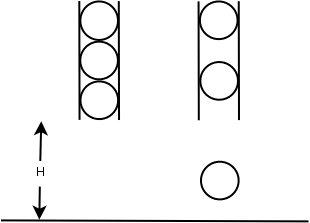# POJ3684 Physics Experiment

## Description

Simon is doing a physics experiment with N identical balls with the same radius of R centimeters. Before the experiment, all N balls are fastened within a vertical tube one by one and the lowest point of the lowest ball is H meters above the ground. At beginning of the experiment, (at second 0), the first ball is released and falls down due to the gravity. After that, the balls are released one by one in every second until all balls have been released. When a ball hits the ground, it will bounce back with the same speed as it hits the ground. When two balls hit each other, they with exchange their velocities (both speed and direction).Simon wants to know where are the N balls after T seconds. Can you help him?

In this problem, you can assume that the gravity is constant: g = 10 m/s2.

## Input

The first line of the input contains one integer C (C ≤ 20) indicating the number of test cases. Each of the following lines contains four integers N, H, R, T. 1≤ N ≤ 100. 1≤ H ≤ 10000 1≤ R ≤ 100 1≤ T ≤ 10000

## Output

For each test case, your program should output N real numbers indicating the height in meters of the lowest point of each ball separated by a single space in a single line. Each number should be rounded to 2 digit after the decimal point.

## Analysis

1. https://dafenghh.github.io/post/d839a1f6.html
2. N 个半径为 R 厘米的球放在 H 米的高度上，下落，球和球、球和地之间弹性碰撞，求 T 秒后各球的高度。
3. 类似蚂蚁相遇问题，球之间碰撞可以忽略。
4. 球的最高上升高度为 H ？
5. 球与球之间的相对位置不会改变。
# POJ